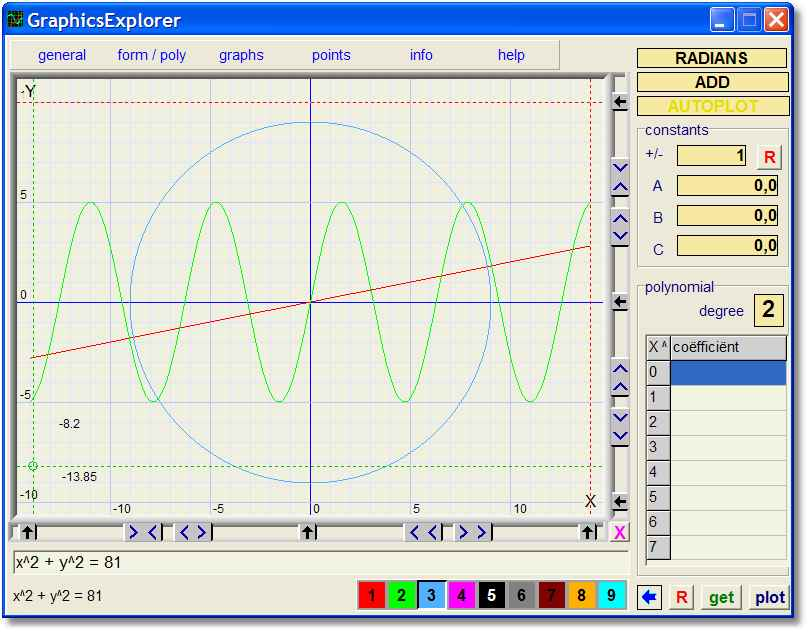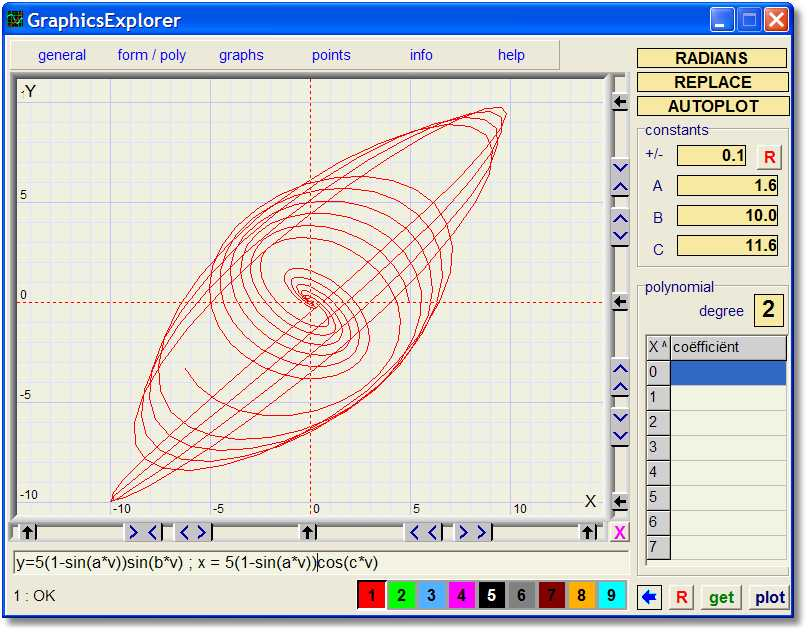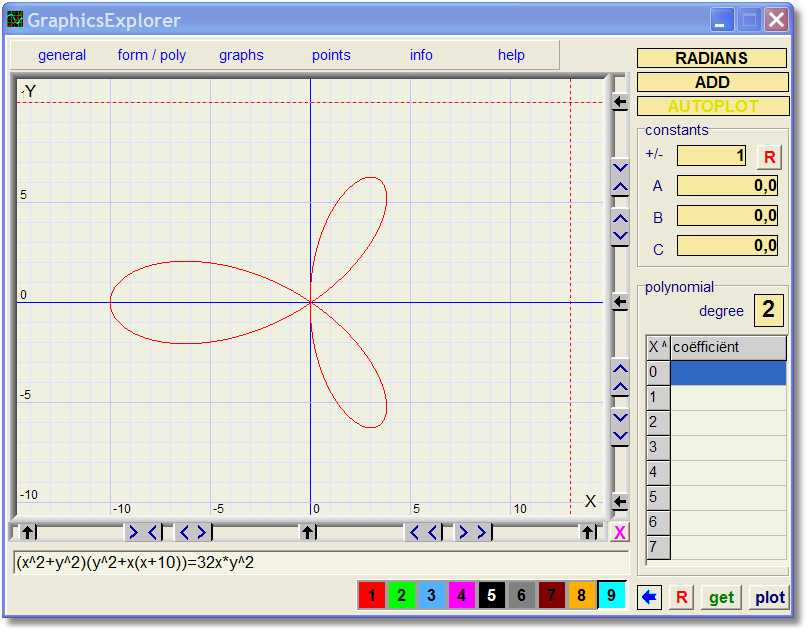Graphics-ExplorerExplore, plot, print, calculate equations and functionsGraphics Explorer version 3 is available, look [ HERE ]
Major changes are
- coordinate system 800*800 pixels
- superscript formula editing: x2 instead of x^2
To see the (Delphi project) source code : look [ HERE ]

Graphics Explorer is a versatile and powerfull program to plot, print or investigate graphs, equations and functions.

Besides the graphing of different type of functions, Graphics Explorer also is able to find
the best fitting polynomial or exponential function given a set of points.

Equations may contain constants (a,b,c) that can be changed by a mouseclick.
Graphs adjust instantly which illustrates the role of constants as in y = a*sin(b*x + c) ,
where a,b,c are amplitude, frequency and shift of phase.

Below is a picture of the program at work.
Just plotted are
y = 0.2x....... y = 5sin(x) ....... x^2 + y^2 = 81Specifications

- coördinate system 600 * 450 pixels
- crosshairs with (x,y) indication
- 9 different colors and formulas
- scrolling and zooming with mouseclick, x/y independent
- add or delete dots with mouseclick or by table editing
- recognizes 4 types of equations:

typeexampledescription
1y = f(x)y = 3cos(5x)function
2x = f(y)x = (y-1)^2inverse
3y = f(v) ; x = g(v)y = 5sin(2v) ; x = 7cos(3v)parametric
4f(x) = g(x)(x^2+y^2)(y^2+x(x+10))=40x*y^2implicit

- enter polynomials, degree 0..7, in "coefficient" table
- operations on polynomials:
• reflections
• integration
• differentiation
• sum, difference, product
- use of constants a,b,c in formula's
- a,b,c changable by mouseclick, graphics adjust automatically
- plot function (type 1) 1..3 times differentiated
by simply preceding it by d:, dd: or ddd: .
example: y = d:sin(2x)
- plot function (type 1) integrated
by simply preceding it by i: .
example: y = i: 5sin(x)
- compute the area under a function
- compute polynomial of degree 0..7 through 1..40 dots.
- compute exponential functions through set of dots  y = a.b^x y = a.b^x + c y = a.e^(bx) y = a.e^(bx) + c y = e^(ax^2 + bx + c)
- all colors adjustable to personal preference.
- add personal heading to plots and prints.
- save / reload user settings
- save / reload formulas
- copy graphic image to clipboard
- print graphics
• 7 sizes
• black / white or colored
• with / without backgroundcolor
- mouse- or keyboard operation
- in line help documentation
- 484kB (.exe) file
- for Windows 95 and up
- screen minimal 800*600 pixels
- no installation procedure, no changes in Windows Registry.

Examples

1. Polynomial (degree 3) given 4 points:2. Lissajous figure, use of constants a,b,c3. Implicit function (trifolium)Installation

Programming language is Delphi.OneStat